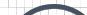Informative Information for the Uninformed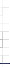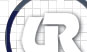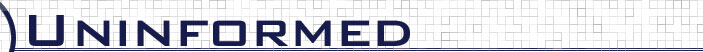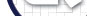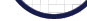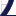Vol 2» 2005.Sept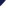# Determining Per-byte Durations

Once the update period and size of a temporal address have been determined, it is possible to calculate the amount of time it takes to change each byte position in the temporal address. For instance, if a four byte temporal address with an update period of 1 second were found in memory, the first byte (or LSB) would change once every second, the second byte would change once every 256 seconds, the third byte would change once every 65536 seconds, and the fourth byte would change once every 16777216 seconds. The reason these properties are exhibited is because each byte position has 256 possibilities (0x00 to 0xff inclusive). This means that each byte position increases in duration by 256 to a given power. This can be described as shown in figure. Let x equal the byte index starting at zero for the LSB.

 duration(x) = 256 ^ x

The next step to take after determining period-specific byte durations is to convert the durations to a measure more aptly accessible assuming a period that is more granular than a second. For instance, figureshows that if each byte duration is measured in 100 nanosecond intervals for an 8 byte temporal address, a conversion can be applied to convert from 100 nanosecond intervals for a byte duration to seconds.

 tosec(x) = duration(x) / 10 ^ 7

This phase is especially important when it comes to calculating viable opcode windows because it is necessary to know for how long a viable opcode will exist which is directly dependent on the direction of the opcode byte closest to the LSB. This will be discussed in more detail in chapter.